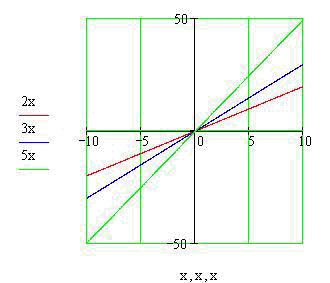Calculus on Demand at Dartmouth College Lecture 9 | Index | Lecture 11 Lecture 10Resources

Math 3 Course Syllabus
Practice Exams

Contents

In this lecture we compute (using limits) the derivatives of polynomial functions and functions related to them. We will find that these computations lead to simple rules and formulas that we can remember easily.

Quick Question

What is the value of the slope of the line whose graph is that of the sum of the two linear functions 2x and 3x?Outline

Outline for Differentiation Rules

Textbook

Differentiation Rules